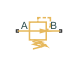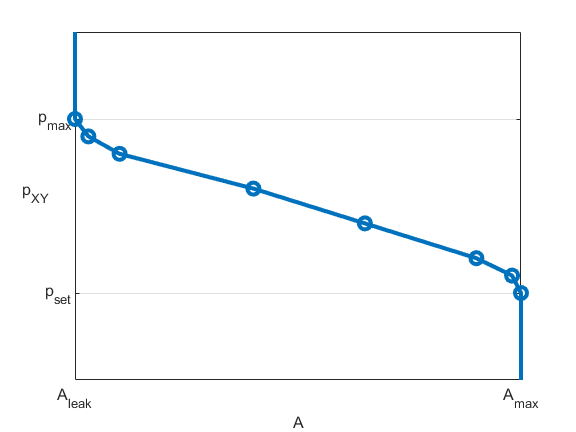# Pressure Reducing Valve (TL)

Pressure reducing valve in a thermal liquid network

•Libraries:
Simscape / Fluids / Thermal Liquid / Valves & Orifices / Pressure Control Valves

## Description

The Pressure Reducing Valve (TL) block represents a valve that reduces downstream pressure in a thermal liquid network. The valve remains open when the pressure at port B is less than a specified pressure. When the pressure at port B meets or surpasses this pressure, the valve closes. The block operates based on the differential between the set pressure and the pressure at port B.

### Mass Balance

The mass conservation equation in the valve is

`${\stackrel{˙}{m}}_{A}+{\stackrel{˙}{m}}_{B}=0,$`

where:

• ${\stackrel{˙}{m}}_{A}$ is the mass flow rate into the valve through port A.

• ${\stackrel{˙}{m}}_{B}$ is the mass flow rate into the valve through port B.

### Mass Flow Rate

The block calculates the mass flow rate as

`$\stackrel{˙}{m}=\frac{{C}_{d}{A}_{valve}\sqrt{2\overline{\rho }}}{\sqrt{P{R}_{loss}\left(1-{\left(\frac{{A}_{valve}}{{A}_{port}}\right)}^{2}\right)}}\frac{\Delta p}{{\left[\Delta {p}^{2}+\Delta {p}_{crit}^{2}\right]}^{1/4}},$`

where:

• Cd is the value of the Discharge coefficient parameter.

• Avalve is the instantaneous valve open area.

• Aport is the value of the Cross-sectional area at ports A and B parameter.

• $\overline{\rho }$ is the average fluid density.

• Δp is the valve pressure difference pApB.

The critical pressure difference, Δpcrit, is the pressure differential associated with the Critical Reynolds number, Recrit, the flow regime transition point between laminar and turbulent flow:

`$\Delta {p}_{crit}=\frac{\pi }{8{A}_{valve}\overline{\rho }}{\left(\frac{\mu {\mathrm{Re}}_{crit}}{{C}_{d}}\right)}^{2},$`

where μ is the dynamic viscosity of the thermal liquid.

The pressure loss, PRloss, describes the reduction of pressure in the valve due to a decrease in area. The block calculates PRloss as

`$P{R}_{loss}=\frac{\sqrt{1-{\left(\frac{{A}_{valve}}{{A}_{port}}\right)}^{2}\left(1-{C}_{d}^{2}\right)}-{C}_{d}\frac{{A}_{valve}}{{A}_{port}}}{\sqrt{1-{\left(\frac{{A}_{valve}}{{A}_{port}}\right)}^{2}\left(1-{C}_{d}^{2}\right)}+{C}_{d}\frac{{A}_{valve}}{{A}_{port}}}.$`

The pressure recovery describes the positive pressure change in the valve due to an increase in area. When you clear the Pressure recovery check box, the block sets PRloss to 1.

### Opening Parameterization

When you set the Opening parameterization parameter to `Linear - Area vs. pressure`, the block calculates the opening area as

`${A}_{valve}=\stackrel{^}{p}\left({A}_{leak}-{A}_{max}\right)+{A}_{max},$`

where Aleak is the value of the Leakage Area parameter and Amax is the value of the Maximum opening area parameter. The normalized pressure, $\stackrel{^}{p}$, is

`$\stackrel{^}{p}=\frac{{p}_{control}-{p}_{set}}{{p}_{Max}-{p}_{set}},$`

where

• pset is the valve set pressure:

`${p}_{set}={p}_{set,gauge}+{p}_{Atm},$`

where PAtm is the atmospheric pressure.

• pMax is the maximum pressure

`${p}_{Max}={p}_{set,gauge}+{p}_{range}+{p}_{Atm}.$`

This figure shows how the block controls the opening area using the linear parameterization.When the valve is in a near-open or near-closed position in the linear parameterization, you can maintain numerical robustness in your simulation by adjusting the parameter. If the parameter is nonzero, the block smoothly saturates the control pressure between pset and pmax. For more information, see Numerical Smoothing.

When you set Opening parameterization to `Tabulated data - Area vs. pressure`, the block calculates the opening area as

`${A}_{valve}=tablelookup\left({p}_{control,TLU,ref},{A}_{TLU},{p}_{control},interpolation=linear,extrapolation=nearest\right),$`

where:

• pcontrol,TLU,ref = pTLU + poffset.

• pTLU is the Pressure differential vector parameter.

• poffset is an internal pressure offset that causes the valve to start closing when pcontrol,TLU,ref = pset.

• ATLU is the Opening area vector parameter.

This figure shows how the block controls the opening area when Opening parameterization is ```Tabulated data - Area vs. pressure```.When you set Opening parameterization to `Tabulated data - Volumetric flow rate vs. pressure`, the valve opens according to the user-provided tabulated data of volumetric flow rate and pressure differential between ports A and B.

The block calculates the mass flow rate as

`$\stackrel{˙}{m}=\overline{\rho }\stackrel{˙}{V},$`

where:

• $\stackrel{˙}{V}$ is the volumetric flow rate.

• $\overline{\rho }$ is the average fluid density.

The block calculates the relationship between the mass flow and pressure using

`$\stackrel{˙}{m}=K\overline{\rho }\sqrt{\Delta p},$`

where

`$\text{K=}\frac{\stackrel{˙}{V}}{\sqrt{|\Delta p|}}.$`

### Opening Dynamics

When you select Opening dynamics, the block introduces a control pressure lag where pcontrol becomes the dynamic control pressure, pdyn. The instantaneous change in dynamic opening area is calculated based on the Opening time constant parameter, τ:

`${\stackrel{˙}{p}}_{dyn}=\frac{{p}_{control}-{p}_{dyn}}{\tau }.$`

By default, the block clears the Opening dynamics check box.

### Energy Balance

The energy conservation equation in the valve is

`${\varphi }_{A}+{\varphi }_{B}=0,$`

where:

• ϕA is the energy flow rate into the valve through port A.

• ϕB is the energy flow rate into the valve through port B.

## Ports

### Conserving

expand all

Thermal liquid conserving port associated with valve inlet A.

Thermal liquid conserving port associated with valve inlet B.

## Parameters

expand all

Method of modeling the opening of the valve. When you set this parameter to `Linear - Area vs. pressure` or `Tabulated data - Area vs. pressure`, the block relates the opening area to the control pressure. When you set this parameter to ```Tabulated data - Volumetric flow rate vs. pressure```, the block takes the reference flow rate and pressure differential curve of the reducing valve.

Pressure at which the valve begins to shut. The opening area remains variable throughout the pressure regulation range of the valve.

Pressure interval over which the valve opening area varies. The interval begins at the value of the Set pressure (gauge) parameter.

#### Dependencies

To enable this parameter, set Opening parameterization to ```Linear - Area vs. pressure```.

Opening area of the valve in the fully open position due to sealing imperfections, when the valve is at the lower limit of the pressure regulation range. The block uses this parameter to scale the valve opening throughout the pressure regulation range.

#### Dependencies

To enable this parameter, set Opening parameterization to ```Linear - Area vs. pressure```.

Sum of all gaps when the valve is in the fully shut position. The block saturates smaller numbers to this value. This parameter contributes to numerical stability by maintaining continuity in the flow.

#### Dependencies

To enable this parameter, set Opening parameterization to ```Linear - Area vs. pressure```.

Vector of gauge pressure values for the tabulated area parameterization of the valve opening area. The vector elements must correspond one-to-one with the elements in the Opening area vector parameter. The elements must be positive and in ascending order. The block uses linear interpolation between the data points.

#### Dependencies

To enable this parameter, set Opening parameterization to ```Tabulated data - Area vs. pressure```.

Vector of valve opening areas for the tabulated area parameterization of the valve opening area. The vector elements must correspond one-to-one with the elements in the Pressure at Port B (gauge) vector parameter. The elements must be positive and in ascending order. The block uses linear interpolation between the data points.

#### Dependencies

To enable this parameter, set Opening parameterization to ```Tabulated data - Area vs. pressure```.

Constant pressure at port A that forms the basis of the reference curve. This value typically corresponds to the maximum operating pressure of the valve. The pressure at port A can be any value during simulation.

#### Dependencies

To enable this parameter, set Opening parameterization to ```Tabulated data - Volumetric flow rate vs. pressure```.

Vector of monotonically decreasing pressure values at port B in the reference curve as the valve opening area changes. The first element is the reference maximum pressure, which is the pressure where the valve fully shuts. The last element is the reference set pressure.

#### Dependencies

To enable this parameter, set Opening parameterization to ```Tabulated data - Volumetric flow rate vs. pressure```.

Vector of monotonically increasing reference flow rates through the valve. The block uses this curve to generate a flow coefficient for the changing valve area.

#### Dependencies

To enable this parameter, set Opening parameterization to ```Tabulated data - Volumetric flow rate vs. pressure```.

Area at ports A and B, which the block uses in the pressure-flow rate equation that determines the mass flow rate through the valve.

Correction factor that the block uses to account for discharge losses in theoretical flows.

#### Dependencies

To enable this parameter, set Opening parameterization to ```Linear - Area vs. pressure``` or ```Tabulated data - Area vs. pressure```.

Upper Reynolds number limit for laminar flow through the valve.

#### Dependencies

To enable this parameter, set Opening parameterization to ```Linear - Area vs. pressure``` or ```Tabulated data - Area vs. pressure```.

Continuous smoothing factor that introduces a layer of gradual change to the flow response when the valve is in near-open or near-closed positions. Set this value to a nonzero value less than one to increase the stability of your simulation in these regimes.

#### Dependencies

To enable this parameter, set Opening parameterization to ```Linear - Area vs. pressure```.

Whether to capture the increase in pressure when fluid flows from a region of smaller cross-sectional area to a region of larger cross-sectional area.

#### Dependencies

To enable this parameter, set Opening parameterization to ```Linear - Area vs. pressure``` or ```Tabulated data - Area vs. pressure```.

Whether to approximate the transient effects to the fluid system due to valve opening. Selecting Opening dynamics approximates opening conditions by introducing a first-order lag in the flow response.

Time constant by which to compute the lag in the opening dynamics.

#### Dependencies

To enable this parameter, select Opening dynamics.

## Version History

Introduced in R2016a

expand all